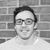# QlikView App Dev

Discussion Board for collaboration related to QlikView App Development.

Announcements
Join this live chat April 6, 10AM EST - QlikView to Qlik Sense REGISTER
cancel
Showing results for
Search instead for
Did you mean:Not applicable

## Use if condition on a set analysis

I have a set analysis set up and was wondering if I could make an if statement out of the set analysis.  Basically I want an if condition that checks to see if the value from the set analysis is greater than 0.

here's the set analysis:

sum({<FYEAR={\$(vBenchYr)},QUARTER={'Q4'},Measure={'Shipped Qty'}>}(Value))

so if the sum of this set analysis value is greater then 0 then I want to do something

how would I do this if it can be done????

3 RepliesMVP

if (sum({<FYEAR={\$(vBenchYr)},QUARTER={'Q4'},Measure={'Shipped Qty'}>}(Value))>0,   '>0',   '<=0')Specialist III

if (sum({<FYEAR={\$(vBenchYr)},QUARTER={'Q4'},Measure={'Shipped Qty'}>}(Value)) >0,

'A','B')Luminary

if ( sum({<FYEAR={\$(vBenchYr)},QUARTER={'Q4'},Measure={'Shipped Qty'}>}(Value)) > 0 , TRUE , FALSE )